# Cirio Mr. Karate Hien Shippuu Kyaku problem (Read 25701 times)

Started by tehdevil, May 03, 2018, 06:18:07 am

#### tehdevil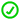##### Cirio Mr. Karate Hien Shippuu Kyaku problem
#1  May 03, 2018, 06:18:07 am
••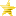Hi, I came across this Mr. Karate created by Cirio's problem which the character can be found here: http://cirio.piiym.net/ it was his strong version of Hien Shippuu Kyaku where he can't connect the 2nd hit on his opponent like this: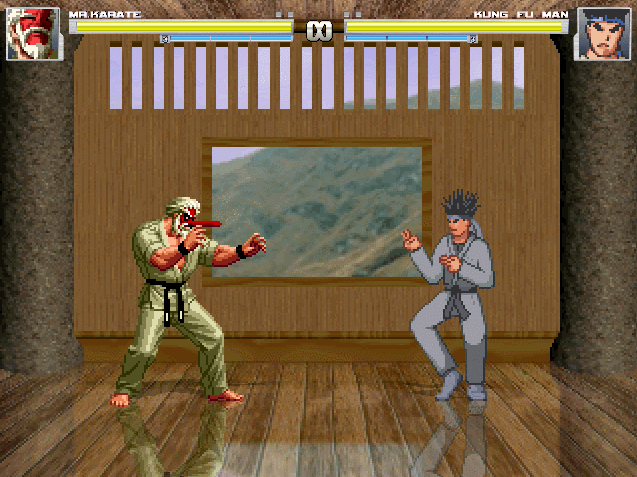it only work when it is a mirror match: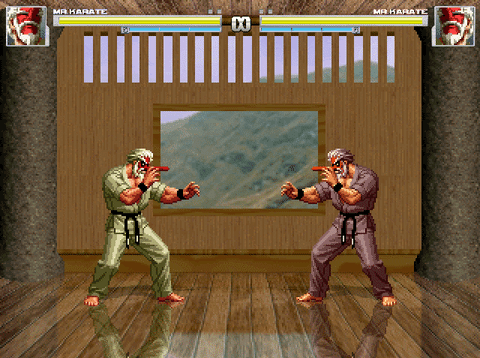Here is the code of the move of this character:
Code:
`;Hien Shippuu Kyaku (Strong)[Statedef 1510]type = Smovetype = Aphysics = Sanim = 1510ctrl = 0velset = 0, 0poweradd = 142juggle = 0[State 2810, snd1]type = PlaySndtrigger1 = Time = 2value = 3,4channel = 0[State 2801,1]type = ChangeStatetrigger1 = AnimTime = 0value = 1511;Hien Shippuu Kyaku Air[Statedef 1511]type = Amovetype = Aphysics = Nanim = 1511velset = 8, 0[State 2000, eff]type = PlaySndtrigger1 = time = 0value = 200,8channel = 2[State 1110,0]type = Posaddtrigger1 = Time = 0trigger2 = Time = 2trigger3 = Time = 3trigger4 = Time = 4trigger5 = Time = 6y = -1[State 1110,0]type = Posaddtrigger1 = Time = 15trigger2 = Time = 17trigger3 = Time = 18trigger4 = Time = 20trigger5 = Time = 23y = 1[State 2820,1]type = HitDeftrigger1 = (p2stateno != [5020,5040])  trigger1 = p2stateno != [154,155]trigger1 = animelem = 2attr = A, SAanimtype = Hardhitflag = MAguardflag = Mdamage = 50, 10hitsound = S250,0guardsound = S201, 2sparkno = S1041guard.sparkno = S1042sparkxy = 17,-68pausetime = 10,12guard.pausetime = 11,15ground.type = Lowground.slidetime = 17ground.hittime = 17ground.velocity = -4,-9air.velocity = -4,-6guard.velocity = -9fall.recovertime = 35guard.slidetime = 16guard.hittime = 11getpower = 50,40givepower = 30,10yaccel = .55fall.yvelocity = -3.4fall = 1[State 2820,2]type = ChangeStatetrigger1 = MoveContact = 1value = 1513[State 2820,3]type = ChangeStatetrigger1 = AnimTime = 0trigger1 = MoveContact = 0value = 1512`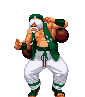<- I exercise and dance like that regularly!
Last Edit: April 27, 2019, 12:08:59 pm by tehdevil

#### Odb718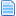##### Re: Cirio Mr. Karate Hien Shippuu Kyaku problem
#2  May 03, 2018, 09:02:39 am
••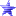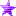• Shame on you!
Use Ctrl+C to see the collision boxes. Make sure they don't connect.
You'll want to adjust the Y velocities set in the hitdef. You could also adjust the Animation so the hit box extends a little higher. Up to you.

#### tehdevil##### Re: Cirio Mr. Karate Hien Shippuu Kyaku problem
#3  April 27, 2019, 12:08:47 pm
••Hi, sorry to revive this, but I found a way to fix the problem, it was this piece of code that cause the problem:

Code:
`;Hien Shippuu Kyaku Air[Statedef 1511]type = Amovetype = Aphysics = Nanim = 1511velset = 8, 0[State 2000, eff]type = PlaySndtrigger1 = time = 0value = 200,8channel = 2[State 1110,0]type = Posaddtrigger1 = Time = 0trigger2 = Time = 2trigger3 = Time = 3trigger4 = Time = 4trigger5 = Time = 6y = -1[State 1110,0]type = Posaddtrigger1 = Time = 15trigger2 = Time = 17trigger3 = Time = 18trigger4 = Time = 20trigger5 = Time = 23y = 1[State 2820,1]type = HitDeftrigger1 = (p2stateno != [5020,5040])  trigger1 = p2stateno != [154,155]trigger1 = animelem = 2attr = A, SAanimtype = Hardhitflag = MAguardflag = Mdamage = 50, 10hitsound = S250,0guardsound = S201, 2sparkno = S1041guard.sparkno = S1042sparkxy = 17,-68pausetime = 10,12guard.pausetime = 11,15ground.type = Lowground.slidetime = 17ground.hittime = 17ground.velocity = -4,-9air.velocity = -4,-6guard.velocity = -9fall.recovertime = 35guard.slidetime = 16guard.hittime = 11getpower = 50,40givepower = 30,10yaccel = .55fall.yvelocity = -3.4fall = 1[State 2820,2]type = ChangeStatetrigger1 = MoveContact = 1value = 1513[State 2820,3]type = ChangeStatetrigger1 = AnimTime = 0trigger1 = MoveContact = 0value = 1512`

so I need to look at this block of code:

Code:
`[State 2820,1]type = HitDeftrigger1 = (p2stateno != [5020,5040])  trigger1 = p2stateno != [154,155]trigger1 = animelem = 2attr = A, SAanimtype = Hardhitflag = MAguardflag = Mdamage = 50, 10hitsound = S250,0guardsound = S201, 2sparkno = S1041guard.sparkno = S1042sparkxy = 17,-68pausetime = 10,12guard.pausetime = 11,15ground.type = Lowground.slidetime = 17ground.hittime = 17ground.velocity = -4,-9air.velocity = -4,-6guard.velocity = -9fall.recovertime = 35guard.slidetime = 16guard.hittime = 11getpower = 50,40givepower = 30,10yaccel = .55fall.yvelocity = -3.4fall = 1`

all I need to do is to change this line:
Code:
`ground.type = Low`
to this:
Code:
`ground.type = Trip`
and it works. This same applies to Jerzy's Mr. Karate and GoD_Ryu's Mr. Karate as well since they modify Cirio's Mr. Karate for their version.<- I exercise and dance like that regularly!